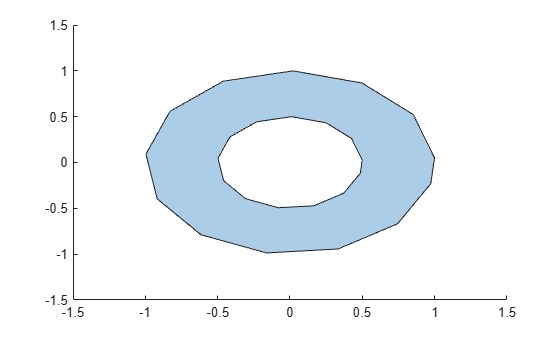# triangulation

Triangulate `polyshape`

## Syntax

``T = triangulation(polyin)``

## Description

example

````T = triangulation(polyin)` returns a 2-D `triangulation` object of a `polyshape` object. `T` has properties that describe the vertices and connectivity of triangles that make up `polyin`. For more information, see `triangulation`.```

## Examples

collapse all

Create and triangulate a polygon.

```t = 0.05:0.5:2*pi; x1 = cos(t); y1 = sin(t); x2 = 0.5*cos(t); y2 = 0.5*sin(t); polyin = polyshape({x1,x2},{y1,y2})```
```polyin = polyshape with properties: Vertices: [27x2 double] NumRegions: 1 NumHoles: 1 ```
`plot(polyin)``T = triangulation(polyin)`
```T = triangulation with properties: Points: [26x2 double] ConnectivityList: [26x3 double] ```
`triplot(T)`## Input Arguments

collapse all

Input `polyshape`, specified as a scalar.

Data Types: `polyshape`